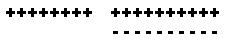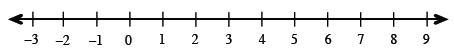### Home > MC2 > Chapter 2 > Lesson 2.1.3 > Problem2-40

2-40.

Simplify the following expressions. Show your work.

1. $8-(-10)$

Represent these values with + - tiles to find out what is left.Notice that the - tiles cancel out and you are left with $18 +$ tiles.

1. $-6-9$

See (a).

$−15$

1. $8-3 + (-4)$Look at the number line. Put your finger at $8$ and move it to the left $3$, then move it to the left $4$. Where do you land?

1. $0-(-3)$

See (a).

1. $15 – 20$

1. $–9 + 14$

See (a) or (e).

1. $11 + \left(–7\right)$

See (a) or (e).

1. $5 – 9$

See (a) or (e).

$−4$